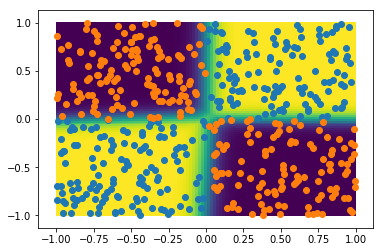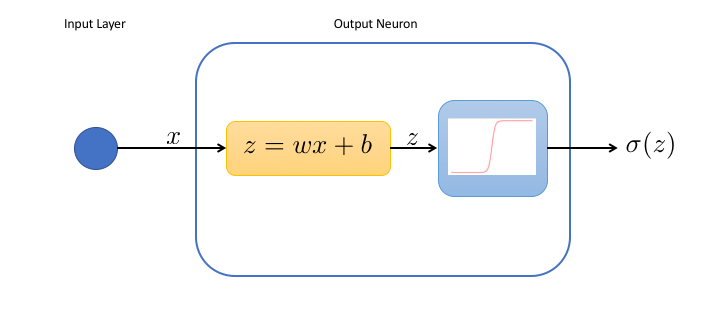# The Data Frog sigmoid## Logistic Regression vs Neural Network: Non Linearities

Back to the logistic regression, aka the 1-neuron network! Here we see that it works well in 2D as well for linear problems. We also find out that it can be necessary to introduce non linearity by adding hidden layers## The 1-Neuron Network: Logistic Regression

So far we've used neural networks as a black box. Today, we're opening the box. To keep it easy, we'll do it for a very, very simple neural network, with a single neuron.

• 1